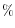## Comparison with the reference sequence and identification of candidate variants

Once we have all of the probabilities for each combination of alleles for all positions in the reference sequence, the next step is to determine which of them have the highest probability of existing in the sample. These are the candidate variations. Nucleotide combinations that are the same as the reference sequence are not reported. At this point in the algorithm, a probability threshold is taken into consideration, utilizing a threshold provided by the user.

The threshold provided by the user indicates how sure one would like to be that the candidate variant differs from the reference type. The threshold is applied by the Probabilistic Variant Caller by considering the inverse situation: is the probability of the candidate variant being the same as the reference position lower than 1 minus the threshold. So, for a user-provided threshold of 90, the Probabilistic Variant Caller requires that any given site type has a probability of less than or equal to 0.1 (i.e. 1 - 0.9) of being the same as the reference type. For example, if a user gave a threshold of 90, and a particular position was found to have a probability of 15, or 0.15, of being the same as the reference (equivalently, having a probability of 85of being different than the reference), then this position would not be called as a variant. If the threshold had been set to 80, then this position would have been called as a variant, as 0.15 is less then 0.20, or in other words, the position has a high enough probability of being different than the reference according to the user-defined threshold, to be reported as a variant.

If a variant is called at a given position, the second step performed by the algorithm is to determines the allele combination (type site) with the highest probability. This type site, together with the corresponding probability, will be reported as the candidate variant.